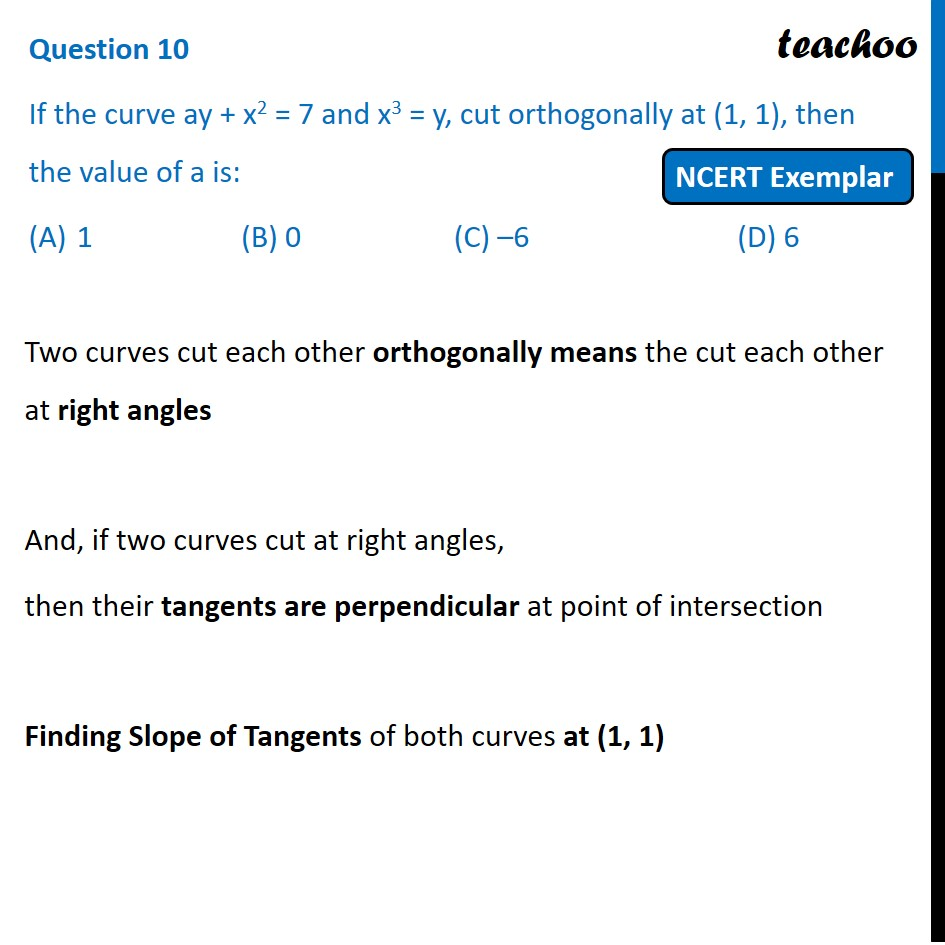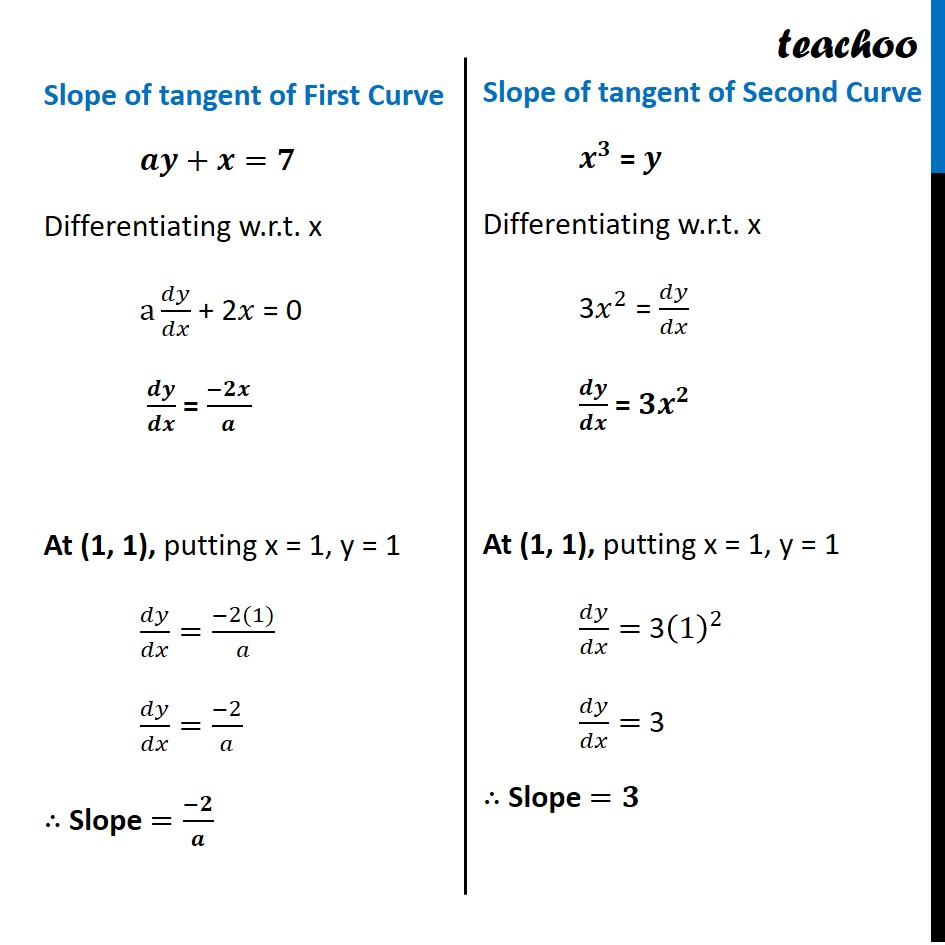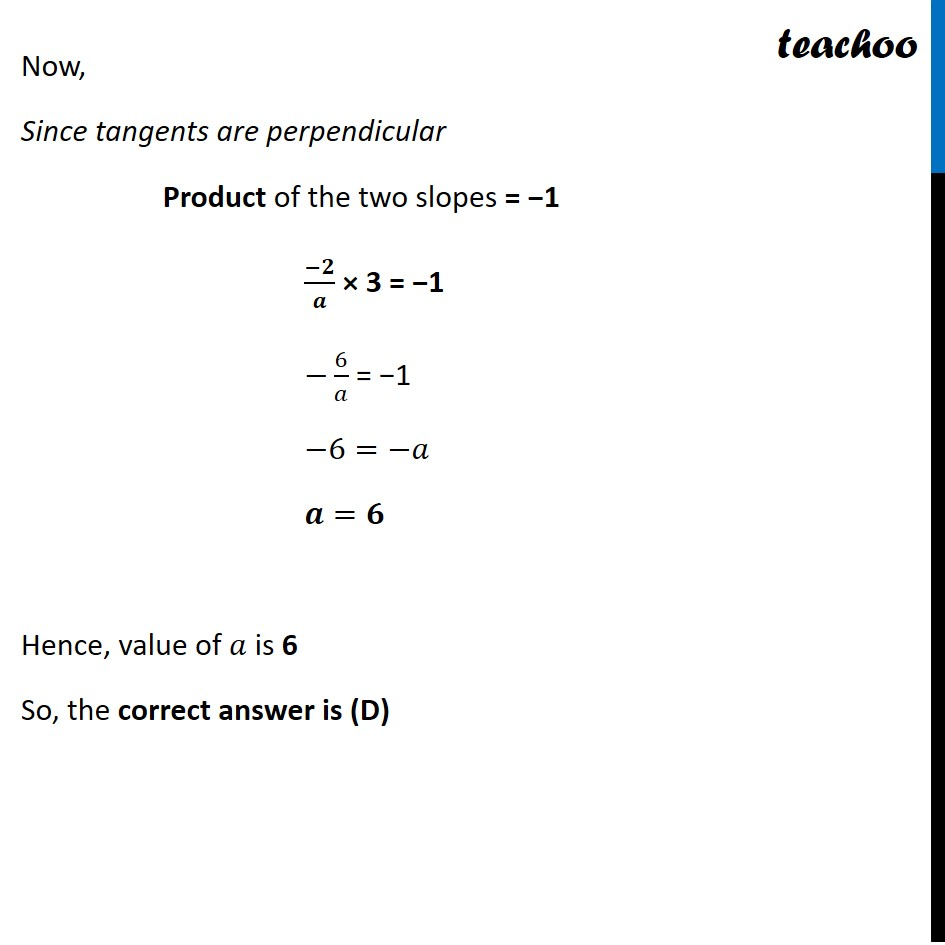NCERT Exemplar - MCQs

Chapter 6 Class 12 Application of Derivatives
Serial order wise

## (A)1   (B) 0   (C) –6   (D) 6Learn in your speed, with individual attention - Teachoo Maths 1-on-1 Class

### Transcript

Question 8 If the curve ay + x2 = 7 and x3 = y, cut orthogonally at (1, 1), then the value of a is: 1 (B) 0 (C) –6 (D) 6 Two curves cut each other orthogonally means the cut each other at right angles And, if two curves cut at right angles, then their tangents are perpendicular at point of intersection Finding Slope of Tangents of both curves at (1, 1) Slope of tangent of First Curve 𝒂𝒚+𝒙=𝟕 Differentiating w.r.t. x a 𝑑𝑦/𝑑𝑥 + 2𝑥 = 0 𝒅𝒚/𝒅𝒙 = (−𝟐𝒙)/𝒂 At (1, 1), putting x = 1, y = 1 𝑑𝑦/𝑑𝑥=(−2(1))/𝑎 𝑑𝑦/𝑑𝑥=(−2)/𝑎 ∴ Slope =(−𝟐)/𝒂 Slope of tangent of Second Curve 𝒙^𝟑 = 𝒚 Differentiating w.r.t. x 3𝑥^2 = 𝑑𝑦/𝑑𝑥 𝒅𝒚/𝒅𝒙 = 𝟑𝒙^𝟐 At (1, 1), putting x = 1, y = 1 𝑑𝑦/𝑑𝑥= 3(1)^2 𝑑𝑦/𝑑𝑥= 3 ∴ Slope =𝟑 Now, Since tangents are perpendicular Product of the two slopes = −1 (−𝟐)/𝒂 × 3 = −1 −6/𝑎 = −1 −6=−𝑎 𝒂=𝟔 Hence, value of 𝑎 is 6 So, the correct answer is (D)